# C# Conditional Statements

## Flow Control and conditional statements

Flow control and conditional statements are available in any programming language to alter the flow of a program.

For example, if someone wants to execute only a particular set of statements based on some certain logic, then Flow control, and conditional statements will be useful.

You will get a better understanding as we go through the various statements which are available in C#.

Please note that all the code below is made to the Program.cs file.

In this tutorial, you will learn-

## 1) If statement

The if statement is used to evaluate a boolean expression before executing a set of statements. If an expression evaluates to true, then it will run one set of statements else it will run another set of statements.

In our example below, a comparison is made for a variable called value. If the value of the variable is less than 10, then it will run one statement, or else it will run on another statement.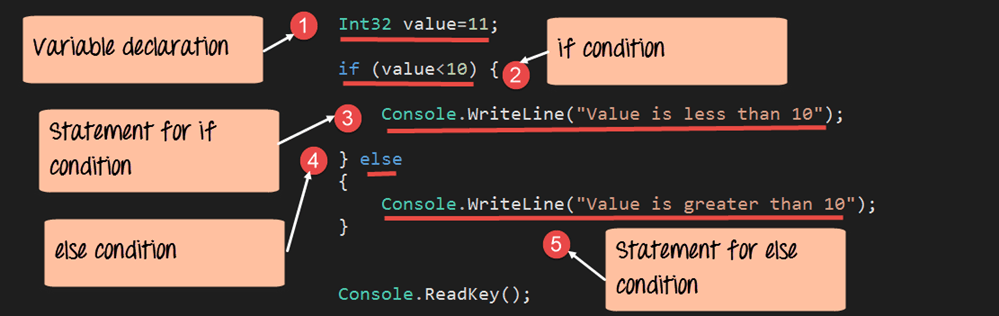```using System;
using System.Collections.Generic;
using System.Linq;
using System.Text;
namespace DemoApplication
{
class Program
{
static void Main(string[] args)
{
Int32 value = 11;

if(value<10)
{
Console.WriteLine("Value is less than 10");
}
else
{
Console.WriteLine("Value is greater than 10");
}
}
}
}```

#### Code Explanation

1. We first define a variable called value and set it to the value of 11.
2. We then use the ‘if’ statement to check if the value is less than 10 of the variable. The result will either be true or false.
3. If the if condition evaluates to true, we then send the message “Value is less than 10” to the console.
4. If the if condition evaluates to false, we then send the message “Value is greater than 10” to the console.

If the above code is entered properly and the program is executed successfully, the following output will be displayed.

#### Output:We can clearly see that the ‘if’ statement was evaluated to false. Hence the message “Value is greater than 10” was sent to the console.

## 2) Switch statement

The switch statement is an enhancement to the ‘if’ statement. If you have multiple expressions that need to be evaluated in one shot, then writing multiple ‘if’ statements becomes an issue.

The switch statement is used to evaluate an expression and run different statements based on the result of the expression. If one condition does not evaluate to true, the switch statement will then move to the next condition and so forth.

Let’s see, how this works with the below example. Here, we are again comparing the value of a variable called ‘value.’ We then check if the value is equal to 1, or 2, or something totally different.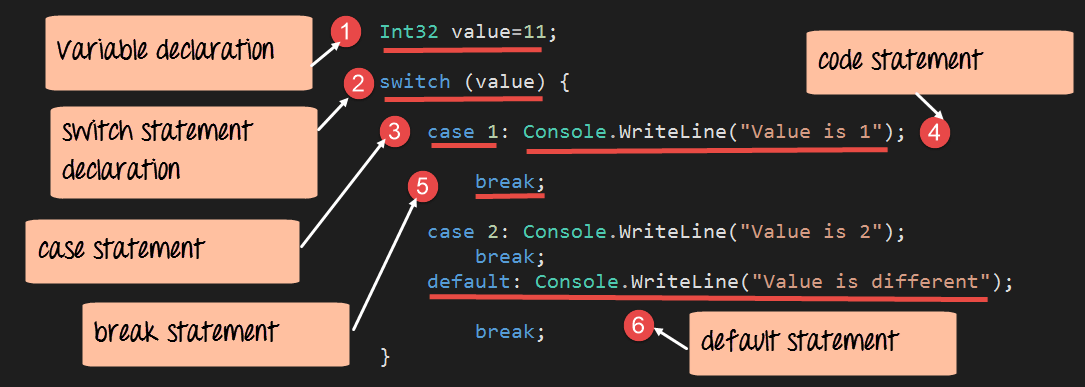```using System;
using System.Collections.Generic;
using System.Linq;
using System.Text;
namespace DemoApplication
{
class Program
{
static void Main(string[] args)
{
Int32 value=11;
switch(value)
{
case 1: Console.WriteLine("Value is 1");
break;
case 2: Console.WriteLine("Value is 2");
break;
default: Console.WriteLine("value is different");
break;
}
}
}
}```

#### Code Explanation:-

1. We first define a variable called ‘value’ and set it to the value of 11.
2. We then use the ‘switch’ statement to check the value of the variable ‘value.’
3. Case statements are used to set different conditions. Based on the conditions, a set of statements can be executed. A switch statement can have multiple case conditions. The first case statement checks to see if the value of the variable is equal to 1.
4. If the first case statement is true, then the message “Value is 1” is written to the console.
5. The break statement is used to break from the entire switch statement, once a condition is true.
6. The default condition is a special condition. This just means that if no case expression evaluates to true, then run the set of statements for the default condition.

If the above code is entered properly and the program is executed successfully, the following output will be displayed. The output prints the default value “Value is different”, since no condition is satisfied.

#### Output: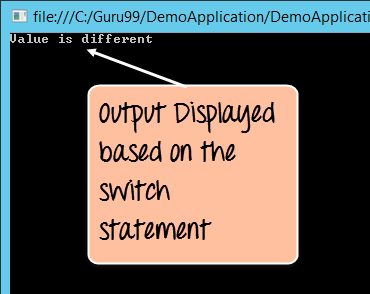## 3) While loop

The while loop is used for iterative purposes. Suppose if you want to repeat a certain set of statements for a particular number of times, then while loop is used.

In our example below, we use the while statement to display the value of a variable ‘i’. The while statement is used to display the value 3 times.```using System;
using System.Collections.Generic;
using System.Linq;
using System.Text;
namespace DemoApplication
{
class Program
{
static void Main(string[] args)
{
Int32 value=3,i=0;

while(i<value)
{
Console.WriteLine(i);
i=i+1;
}
}
}
}```

#### Code Explanation:-

1. Two Integer variables are defined, one being value and the other being ‘i’. The value variable is used as the upper limit to which we should iterate our while statement. And ‘i’ is the variable which will be processed during the iteration.
2. In the while statement, the value of ‘i’ is constantly checked against the upper limit.
3. Here we display the value of ‘i’ to the console. We also increment the value of ‘i’.

If the above code is entered properly and the program is executed successfully, the following output will be displayed.

#### Output: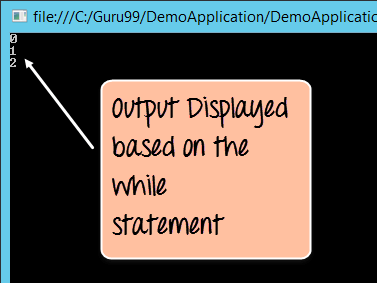Here you can see that the while statement is executed 3 times and incremented at the same time. And each time, it displayed the current value of the variable ‘i’.

## 4) For loop

The ‘for’ loop is also used for iterative purposes. Suppose if you want to repeat a certain set of statements for a particular number of times, then forloop is used.

In our example below, we use the ‘for’ statement to display the value of a variable ‘i’. The ‘for’ statement is used to display the value 3 times.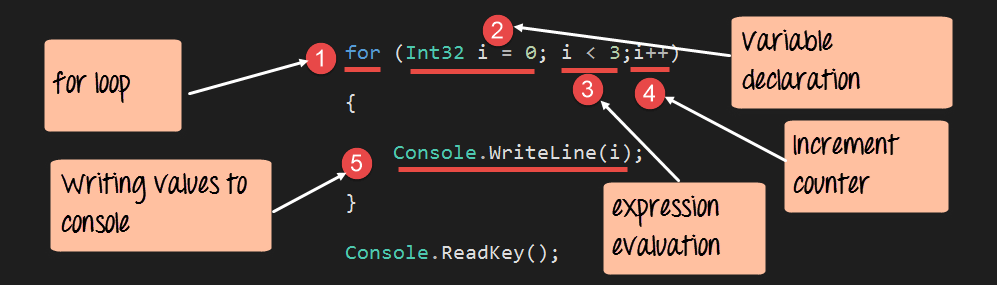```using System;
using System.Collections.Generic;
using System.Linq;
using System.Text;
namespace DemoApplication
{
class Program
{
static void Main(string[] args)
{
for(Int32 i=0;i<3;i++)
{
Console.WriteLine(i);
}

}
}
}```

#### Code Explanation:-

1. The ‘for’ keyword is used to start off the ‘for loop’ statement.
2. In the ‘for loop’, we define 3 things. The first is to initialize the value of a variable, which will be used in the ‘for loop’.
3. The second is to compare the value of the ‘i’ against an upper limit. In our case, the upper limit is the value of 3 (i<3).
4. Finally, we increment the value of ‘i’ accordingly.
5. Here we display the value of ‘i’ to the console.

If the above code is entered properly and the program is executed successfully, the following output will be displayed.

#### Output:Here you can see that the ‘for’ statement is executed 3 times. And each time, it displayed the current value of the variable ‘i’.